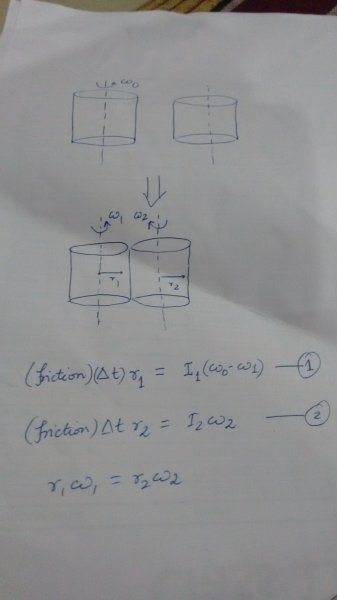# Two cylinders in contact come to final angular velocities

## Homework Statement

Two cylinders, made from the same material and having the same length,have radii r1 and r2with r1> r2. Both are free to rotate about their respective axes. The larger cylinder is initially rotating with angular velocity ωo. The smaller cylinder is moved until it comes into contact withthe larger one. Eventually the frictional force causes both cylinders torotate with constant angular velocity but in opposite directions. Find the final angular velocity of the smaller cylinder. Are any dynamical quantities conserved in this case?

ω1 is the final angular speed of the larger cylinder r1 and ω2 is the final angular speed for the smaller cylinder r2.

## Homework Equations

The angular impulse k is equal to the change in the angular momentum ΔL for both cylinders. k is defined as the time integral of the torque so k=rFt where F is the friction force. Also, L=Iω and r1ω1=r2ω2 at the end.

## The Attempt at a Solution

r1Ft=I11o)
r2Ft=I2ω2
r1ω1=r2ω2
Using these 3 equations I am able to solve for the angular speed of the smaller cylinder (ω2) but my final answer involves the moments of inertia. Am I forgetting something or is this a sufficient answer? Also, no dynamical quantities are conserved due to friction.